# Chapter 4 Atoms and Elements Experiencing Atoms Atoms

• Slides: 57Chapter 4 Atoms and Elements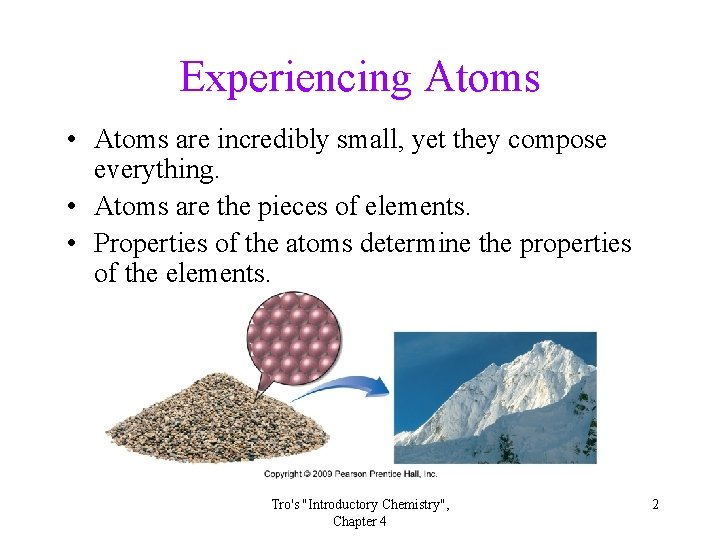Experiencing Atoms • Atoms are incredibly small, yet they compose everything. • Atoms are the pieces of elements. • Properties of the atoms determine the properties of the elements. Tro's "Introductory Chemistry", Chapter 4 2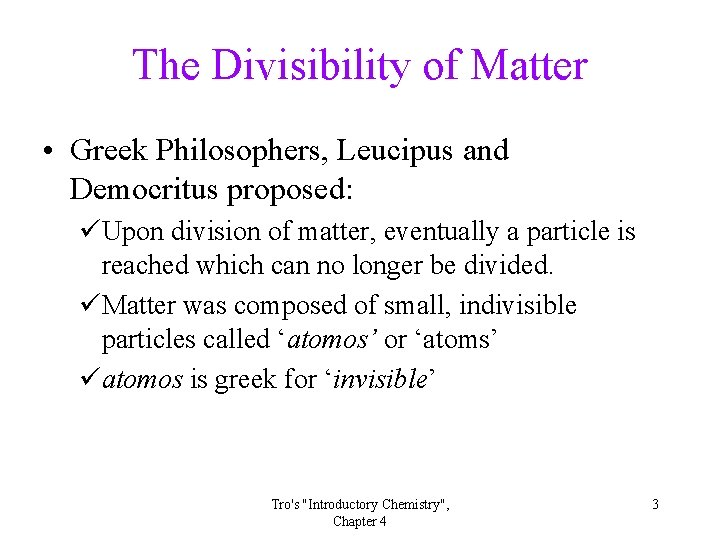The Divisibility of Matter • Greek Philosophers, Leucipus and Democritus proposed: üUpon division of matter, eventually a particle is reached which can no longer be divided. üMatter was composed of small, indivisible particles called ‘atomos’ or ‘atoms’ üatomos is greek for ‘invisible’ Tro's "Introductory Chemistry", Chapter 4 3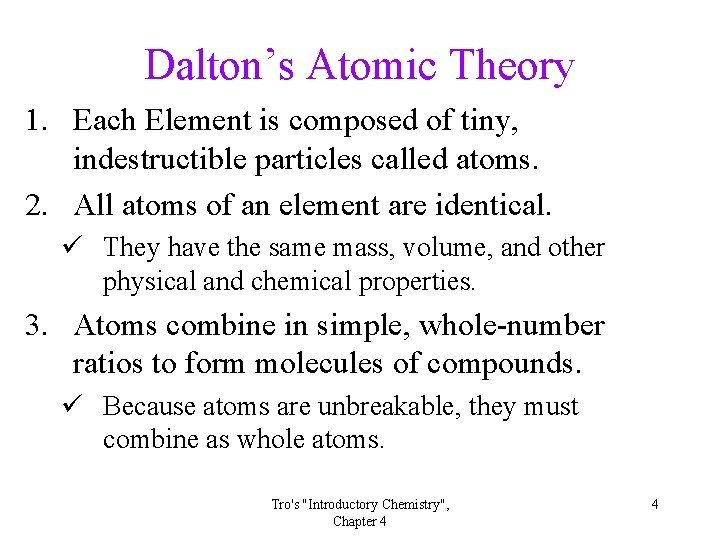Dalton’s Atomic Theory 1. Each Element is composed of tiny, indestructible particles called atoms. 2. All atoms of an element are identical. ü They have the same mass, volume, and other physical and chemical properties. 3. Atoms combine in simple, whole-number ratios to form molecules of compounds. ü Because atoms are unbreakable, they must combine as whole atoms. Tro's "Introductory Chemistry", Chapter 4 4Some Notes on Charges • There are two kinds of charges, called positive and negative. • Opposite charges attract. ü + attracted to –. • Like charges repel. ü + repels +. ü – repels –. • To be neutral, something must have no charge or equal amounts of opposite charges. Tro's "Introductory Chemistry", Chapter 4 5The Atom Is Divisible • Work done by J. J. Thomson proved that the atom had pieces called electrons. • Thomson found that electrons are much smaller than atoms and carry a negative charge. üThe mass of the electron is 1/1836 th the mass of a hydrogen atom. üThe charge on the electron is the fundamental unit of charge that we call – 1 charge unit. Tro's "Introductory Chemistry", Chapter 4 6Thomson’s Model of the Atom 1. The atom is breakable. 2. The atom’s structure has electrons suspended in a positively charged electric field. ü It must have a positive charge to balance a negative charge of electrons. 3. The mass of the atom is due to the mass of the electrons Tro's "Introductory Chemistry", Chapter 4 7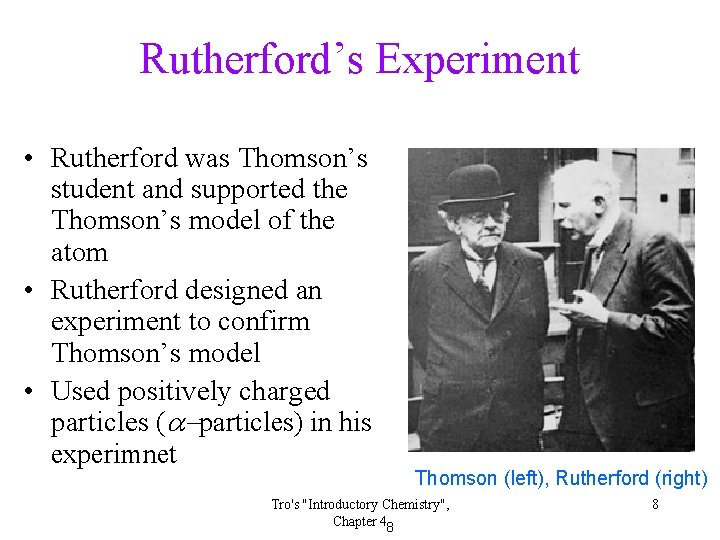Rutherford’s Experiment • Rutherford was Thomson’s student and supported the Thomson’s model of the atom • Rutherford designed an experiment to confirm Thomson’s model • Used positively charged particles (a-particles) in his experimnet Thomson (left), Rutherford (right) Tro's "Introductory Chemistry", Chapter 48 8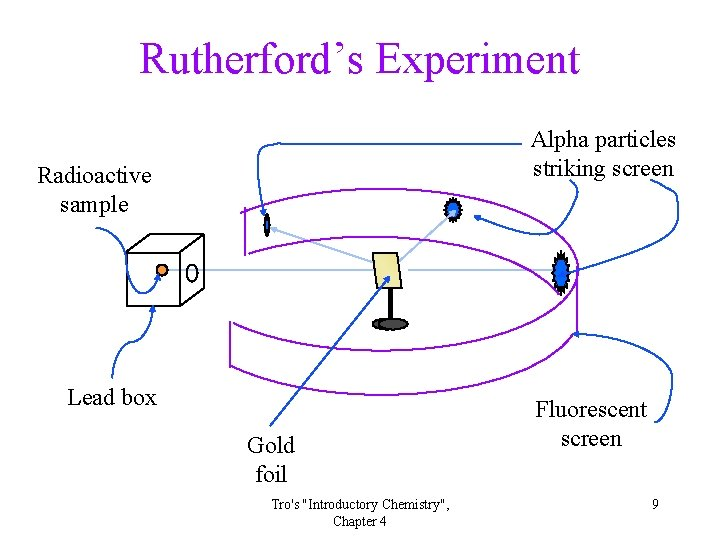Rutherford’s Experiment Alpha particles striking screen Radioactive sample Lead box Gold foil Tro's "Introductory Chemistry", Chapter 4 Fluorescent screen 9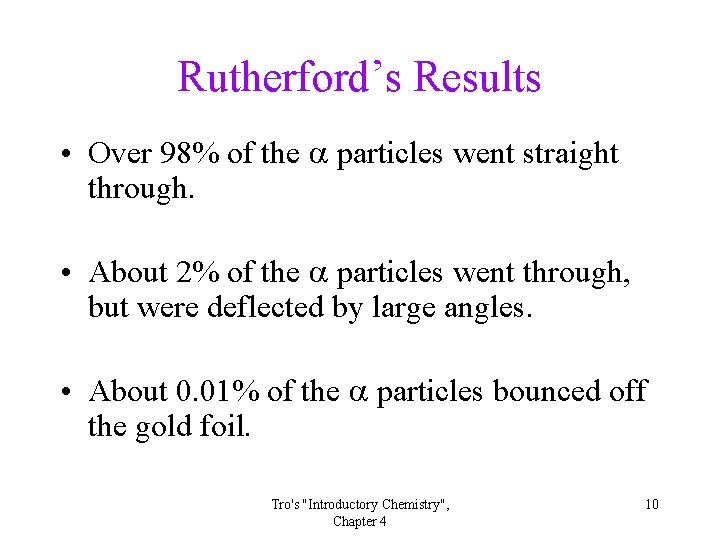Rutherford’s Results • Over 98% of the a particles went straight through. • About 2% of the a particles went through, but were deflected by large angles. • About 0. 01% of the a particles bounced off the gold foil. Tro's "Introductory Chemistry", Chapter 4 10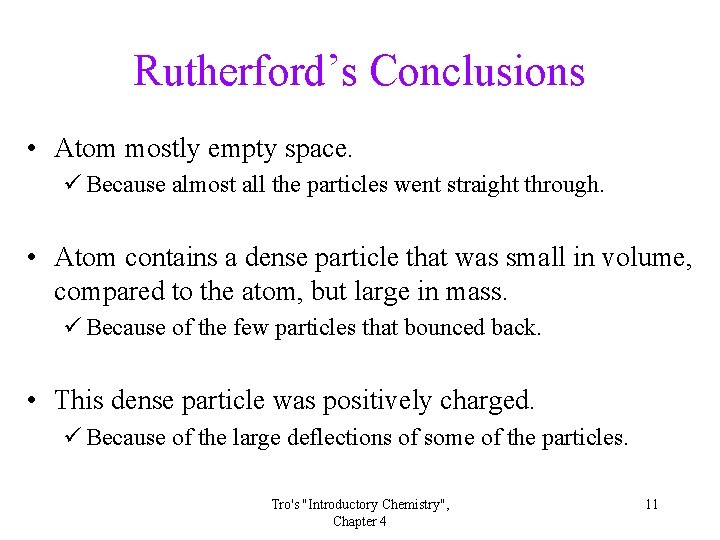Rutherford’s Conclusions • Atom mostly empty space. ü Because almost all the particles went straight through. • Atom contains a dense particle that was small in volume, compared to the atom, but large in mass. ü Because of the few particles that bounced back. • This dense particle was positively charged. ü Because of the large deflections of some of the particles. Tro's "Introductory Chemistry", Chapter 4 11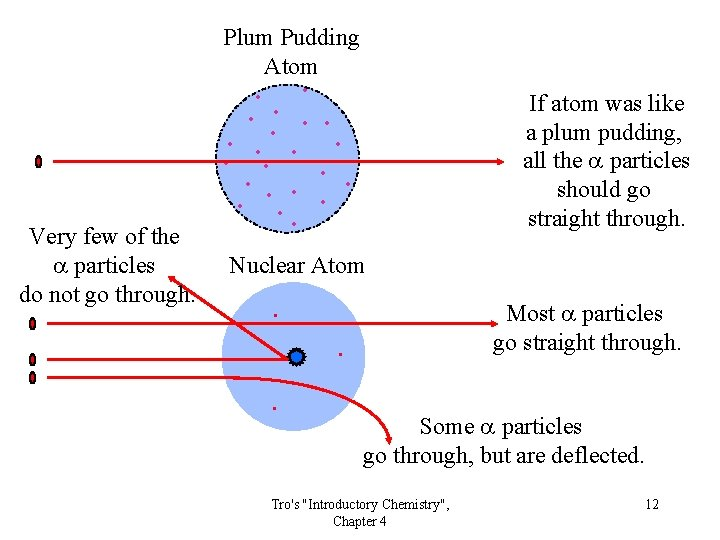Plum Pudding Atom • • • Very few of the a particles do not go through. • • • If atom was like a plum pudding, all the a particles should go straight through. • • • Nuclear Atom . Most a particles go straight through. . . Some a particles go through, but are deflected. Tro's "Introductory Chemistry", Chapter 4 12Rutherford’s Interpretation— The Nuclear Model 1. The atom contains a tiny dense center called the nucleus. ü The amount of space taken by the nucleus is only about 1/10 trillionth the volume of the atom. 2. The nucleus has essentially the entire mass of the atom. ü The electrons weigh so little they contribute practically no mass to the atom. 3. The nucleus is positively charged. ü The amount of positive charge balances the negative charge of the electrons. 4. The electrons are dispersed in the empty space of the atom surrounding the nucleus. ü Like water droplets in a cloud. 13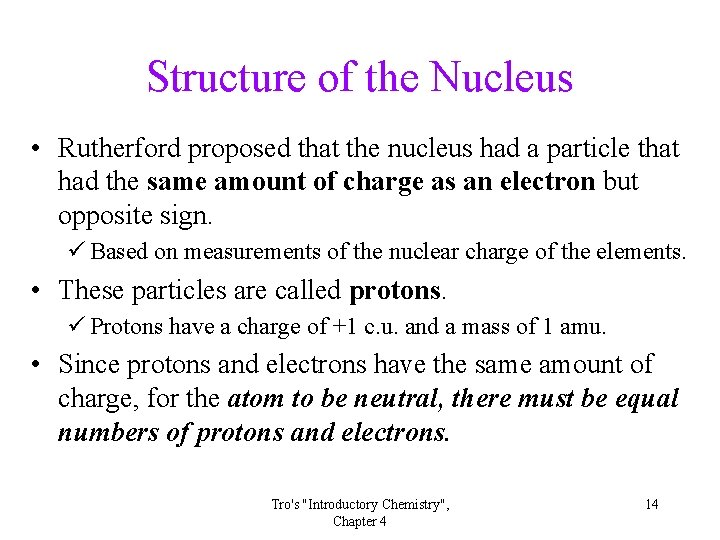Structure of the Nucleus • Rutherford proposed that the nucleus had a particle that had the same amount of charge as an electron but opposite sign. ü Based on measurements of the nuclear charge of the elements. • These particles are called protons. ü Protons have a charge of +1 c. u. and a mass of 1 amu. • Since protons and electrons have the same amount of charge, for the atom to be neutral, there must be equal numbers of protons and electrons. Tro's "Introductory Chemistry", Chapter 4 14Some Problems • How could beryllium have 4 protons stuck together in the nucleus? üShouldn’t they repel each other? • If a beryllium atom has 4 protons, then it should weigh 4 amu, but it actually weighs 9. 01 amu! Where is the extra mass coming from? üEach proton weighs 1 amu. üRemember: The electron’s mass is only about 0. 00055 amu and Be has only 4 electrons—it can’t account for the extra 5 amu of mass. Tro's "Introductory Chemistry", Chapter 4 15There Must Be Something Else • To answer these questions, Rutherford proposed that there was another particle in the nucleus—it is called a neutron. • Neutrons have no charge and a mass of 1 amu. üThe masses of the proton and neutron are both approximately 1 amu. Tro's "Introductory Chemistry", Chapter 4 16The Modern Atom • We know atoms are composed of three main pieces— protons, neutrons, and electrons. • The nucleus is located at the center of the atom and contains protons and neutrons. • The electrons are located outside the nucleus. Tro's "Introductory Chemistry", Chapter 4 17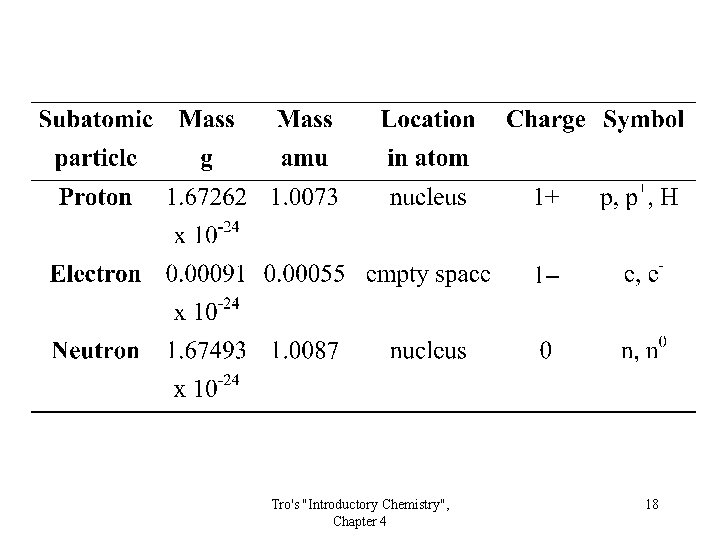Tro's "Introductory Chemistry", Chapter 4 18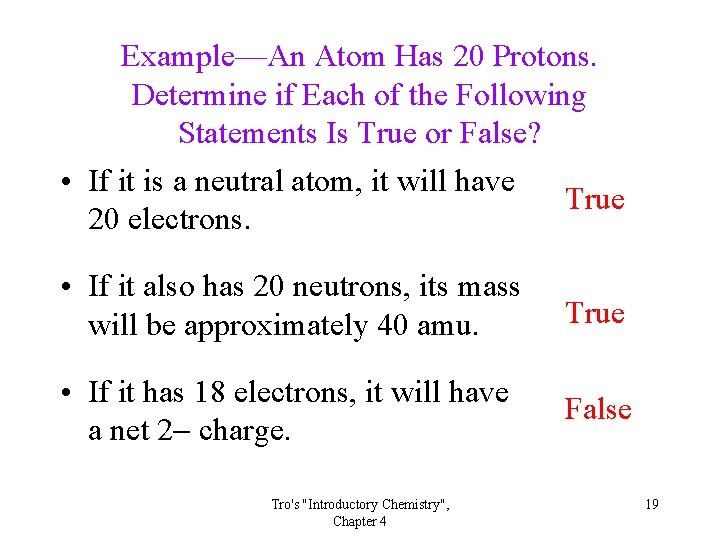Example—An Atom Has 20 Protons. Determine if Each of the Following Statements Is True or False? • If it is a neutral atom, it will have True 20 electrons. • If it also has 20 neutrons, its mass will be approximately 40 amu. True • If it has 18 electrons, it will have a net 2 charge. False Tro's "Introductory Chemistry", Chapter 4 19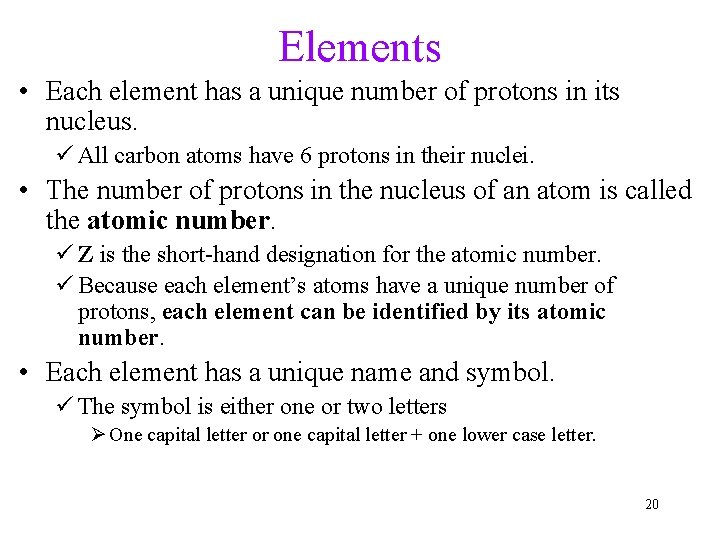Elements • Each element has a unique number of protons in its nucleus. ü All carbon atoms have 6 protons in their nuclei. • The number of protons in the nucleus of an atom is called the atomic number. ü Z is the short-hand designation for the atomic number. ü Because each element’s atoms have a unique number of protons, each element can be identified by its atomic number. • Each element has a unique name and symbol. ü The symbol is either one or two letters Ø One capital letter or one capital letter + one lower case letter. 20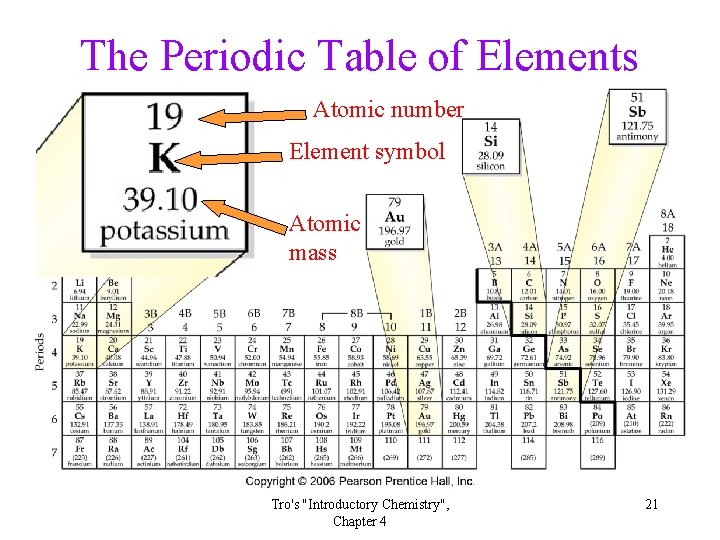The Periodic Table of Elements Atomic number Element symbol Atomic mass Tro's "Introductory Chemistry", Chapter 4 21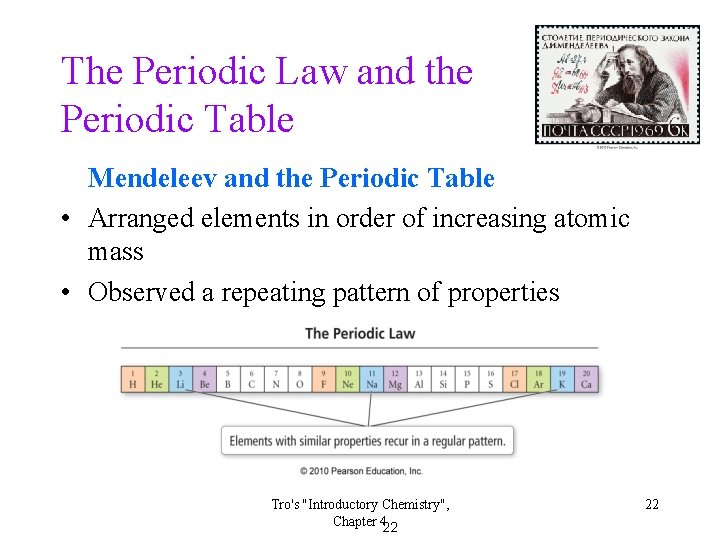The Periodic Law and the Periodic Table Mendeleev and the Periodic Table • Arranged elements in order of increasing atomic mass • Observed a repeating pattern of properties Tro's "Introductory Chemistry", Chapter 422 22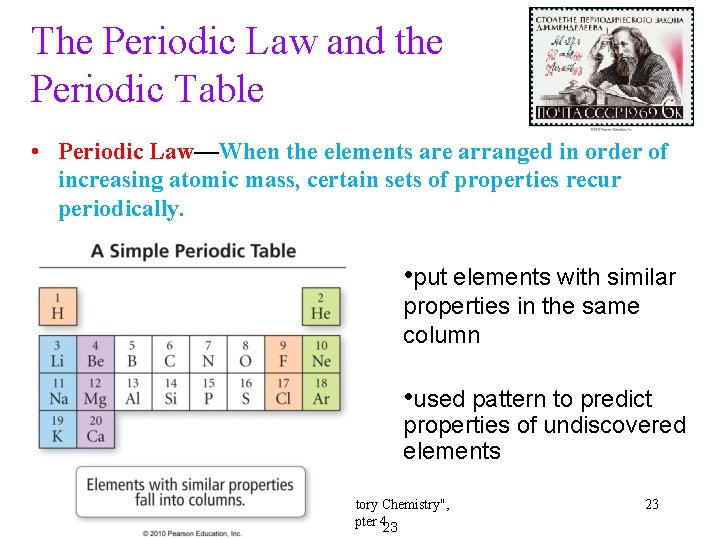The Periodic Law and the Periodic Table • Periodic Law—When the elements are arranged in order of increasing atomic mass, certain sets of properties recur periodically. • put elements with similar properties in the same column • used pattern to predict properties of undiscovered elements Tro's "Introductory Chemistry", Chapter 423 23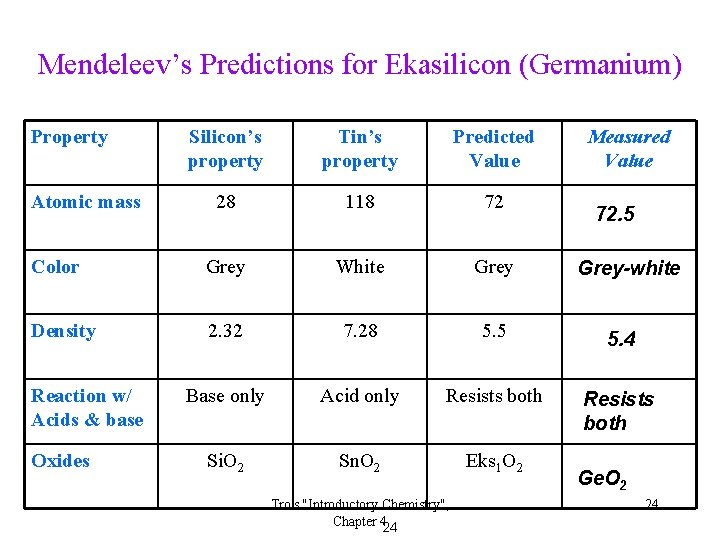Mendeleev’s Predictions for Ekasilicon (Germanium) Property Silicon’s property Tin’s property Predicted Value 28 118 72 Color Grey White Grey Density 2. 32 7. 28 5. 5 5. 4 Base only Acid only Resists both Si. O 2 Sn. O 2 Eks 1 O 2 Atomic mass Reaction w/ Acids & base Oxides Tro's "Introductory Chemistry", Chapter 424 Measured Value 72. 5 Grey-white Ge. O 2 24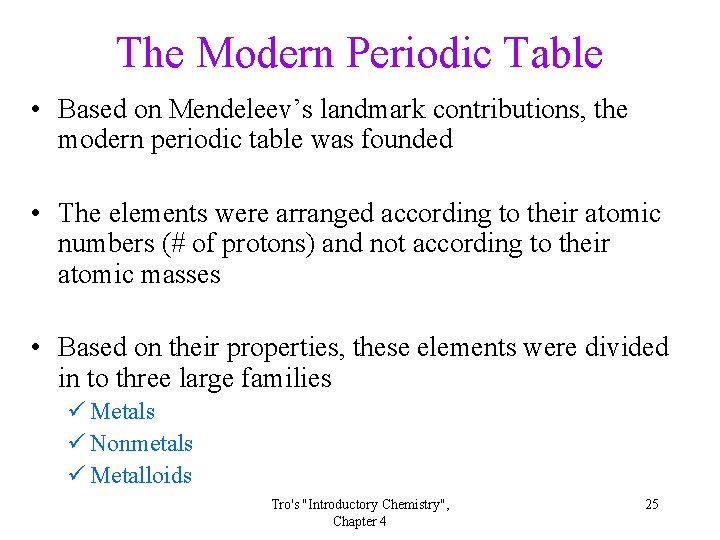The Modern Periodic Table • Based on Mendeleev’s landmark contributions, the modern periodic table was founded • The elements were arranged according to their atomic numbers (# of protons) and not according to their atomic masses • Based on their properties, these elements were divided in to three large families ü Metals ü Nonmetals ü Metalloids Tro's "Introductory Chemistry", Chapter 4 25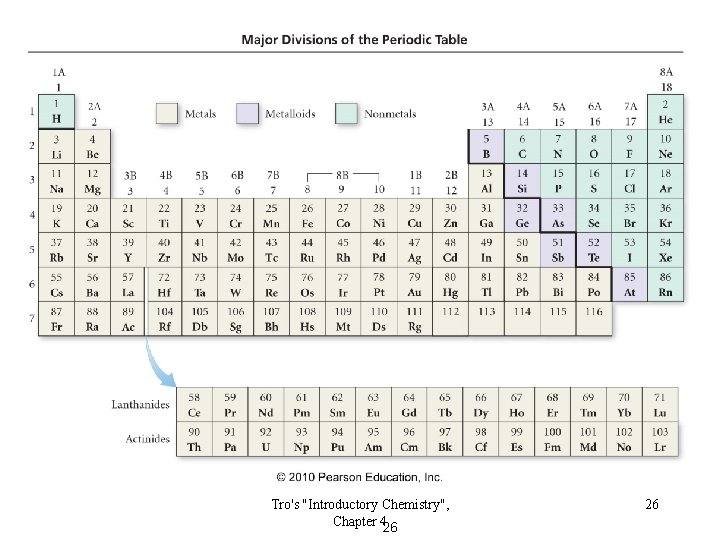Tro's "Introductory Chemistry", Chapter 426 26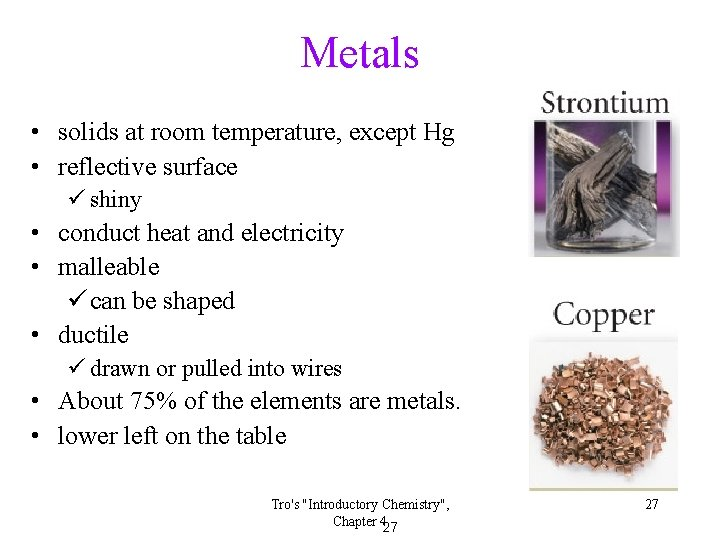Metals • solids at room temperature, except Hg • reflective surface ü shiny • conduct heat and electricity • malleable ü can be shaped • ductile ü drawn or pulled into wires • About 75% of the elements are metals. • lower left on the table Tro's "Introductory Chemistry", Chapter 427 27Nonmetals Sulfur, S(s) • found in all three states • poor conductors of heat Bromine, Br 2(l) • poor conductors of electricity • Solids are brittle. Chlorine, Cl 2(g) • upper right on the table ü except H Tro's "Introductory Chemistry", Chapter 428 28Metalloids • show some properties of metals and some of nonmetals • also known as semiconductors Properties of Silicon ØShiny as a metal ØConducts electricity as a metal ØBrittle as a nonmetal Tro's "Introductory Chemistry", Chapter 429 29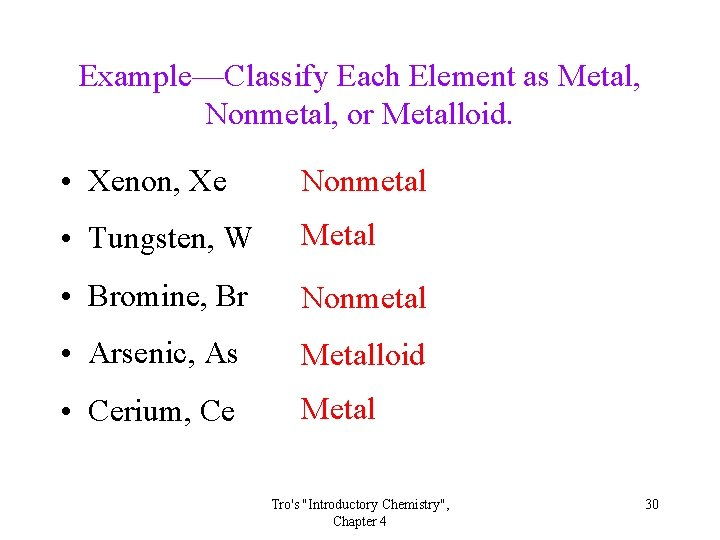Example—Classify Each Element as Metal, Nonmetal, or Metalloid. • Xenon, Xe Nonmetal • Tungsten, W Metal • Bromine, Br Nonmetal • Arsenic, As Metalloid • Cerium, Ce Metal Tro's "Introductory Chemistry", Chapter 4 30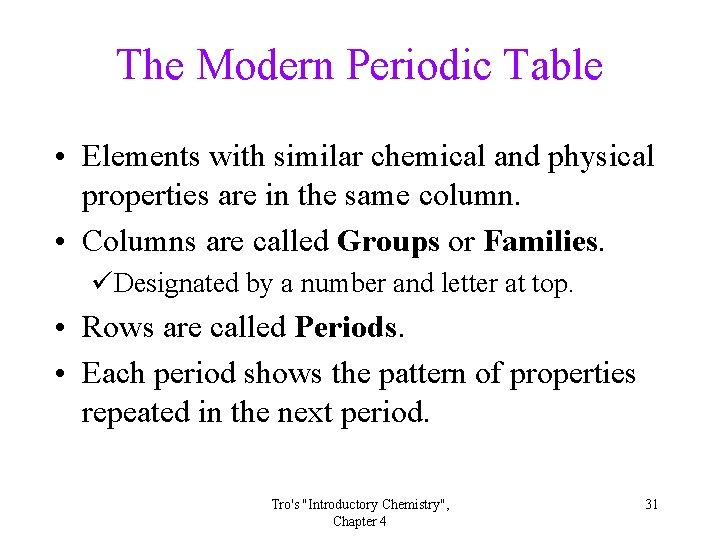The Modern Periodic Table • Elements with similar chemical and physical properties are in the same column. • Columns are called Groups or Families. üDesignated by a number and letter at top. • Rows are called Periods. • Each period shows the pattern of properties repeated in the next period. Tro's "Introductory Chemistry", Chapter 4 31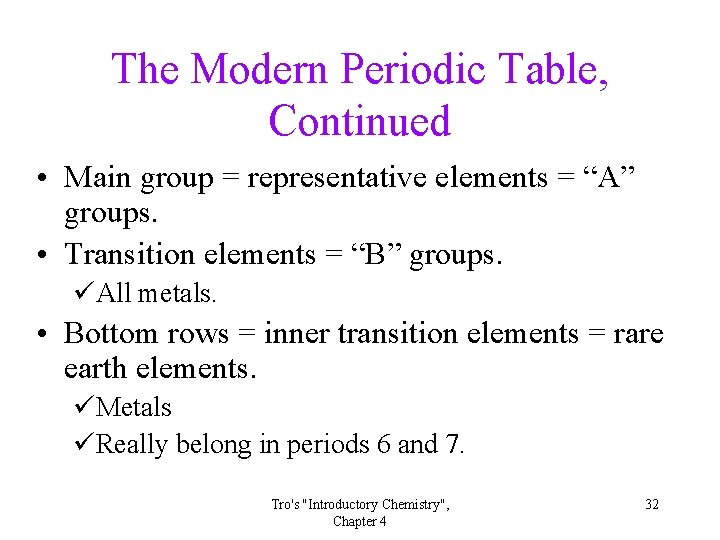The Modern Periodic Table, Continued • Main group = representative elements = “A” groups. • Transition elements = “B” groups. üAll metals. • Bottom rows = inner transition elements = rare earth elements. üMetals üReally belong in periods 6 and 7. Tro's "Introductory Chemistry", Chapter 4 32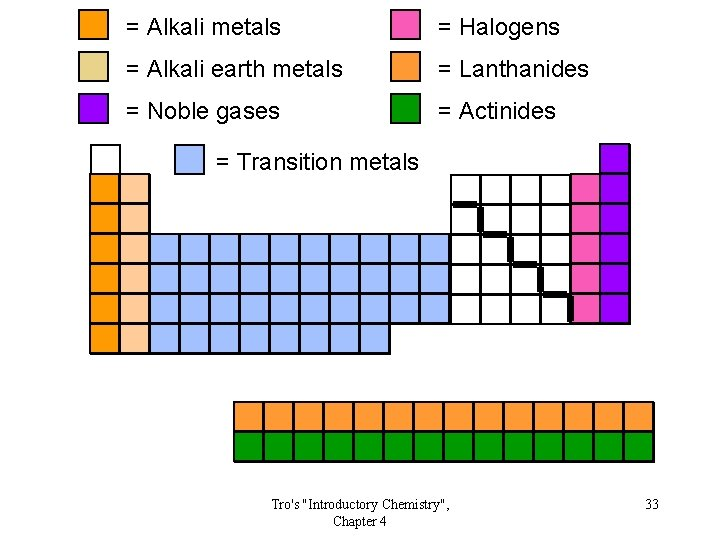= Alkali metals = Halogens = Alkali earth metals = Lanthanides = Noble gases = Actinides = Transition metals Tro's "Introductory Chemistry", Chapter 4 33Important Groups—Hydrogen • Nonmetal. • Colorless, diatomic gas. ü Very low melting point and density. • Reacts with nonmetals to form molecular compounds. ü HCl is an acidic gas. ü H 2 O is a liquid. • Reacts with metals to form hydrides. ü Metal hydrides react with water to form H 2. • hydrogen halides dissolve in water to form acids. Tro's "Introductory Chemistry", Chapter 4 34Group 1 A: Alkali Metals • Hydrogen is usually placed here, though it doesn’t belong. lithium • Soft, low melting points, low density. sodium • Very reactive • Tend to form water soluble compounds potassium ØColorless solutions. • React with water to form basic rubidium (alkaline) solutions and H 2: 2 Na + 2 H 2 O 2 Na. OH + H 2 ØReleases a lot of heat. cesium Tro's "Introductory Chemistry", Chapter 4 35Groups 2 A: Alkaline Earth Metals • Harder, higher melting, and denser than alkali metals. • Reactive, but less than the corresponding group I A metals. • Form stable, insoluble oxides. • Oxides are basic = alkaline earth. • Reactivity with water to form H 2: Be = none, Mg = steam, Ca, Sr, Ba = cold water. beryllium magnesium calcium strontium barium Tro's "Introductory Chemistry", Chapter 4 36Group 7 A: Halogens • Nonmetals. • F 2 and Cl 2 gases, Br 2 liquid, and I 2 solid. • All diatomic. fluorine chlorine bromine iodine • Very reactive. 37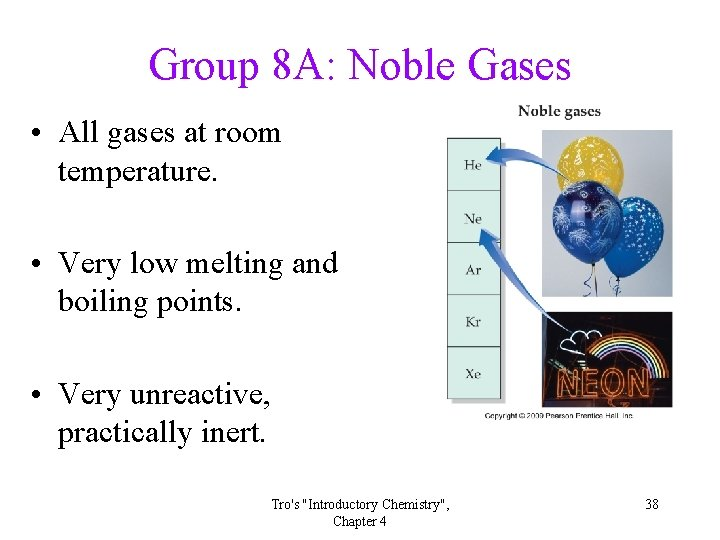Group 8 A: Noble Gases • All gases at room temperature. • Very low melting and boiling points. • Very unreactive, practically inert. Tro's "Introductory Chemistry", Chapter 4 38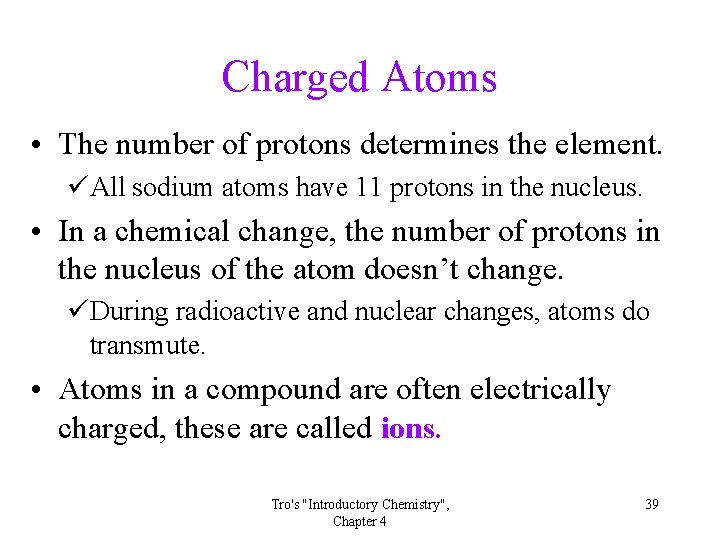Charged Atoms • The number of protons determines the element. üAll sodium atoms have 11 protons in the nucleus. • In a chemical change, the number of protons in the nucleus of the atom doesn’t change. üDuring radioactive and nuclear changes, atoms do transmute. • Atoms in a compound are often electrically charged, these are called ions. Tro's "Introductory Chemistry", Chapter 4 39Ions • Atoms acquire a charge by gaining or losing electrons. ü Not protons! • Ion charge = # protons – # electrons. • Ions with a positive charge are called cations. ü More protons than electrons. ü Form by losing electrons. • Ions with a negative charge are called anions. ü More electrons than protons. ü Form by gaining electrons. • Chemically, ions are much different than the neutral atoms. ü Because they have a different structure. Tro's "Introductory Chemistry", Chapter 4 40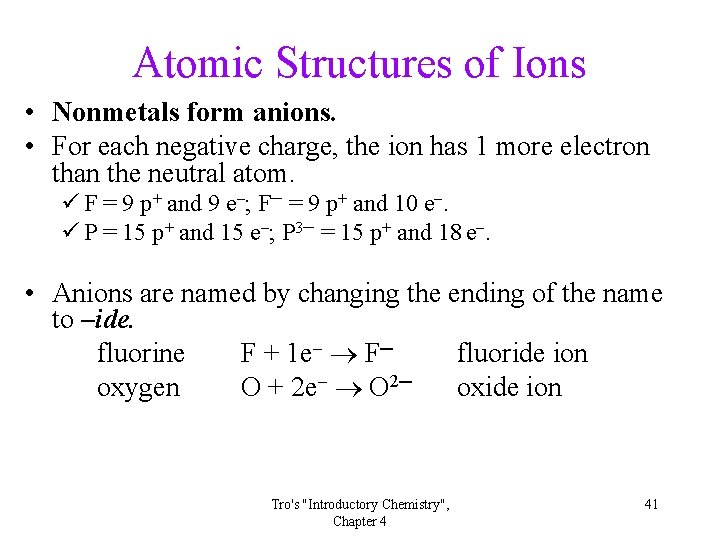Atomic Structures of Ions • Nonmetals form anions. • For each negative charge, the ion has 1 more electron than the neutral atom. ü F = 9 p+ and 9 e ; F─ = 9 p+ and 10 e. ü P = 15 p+ and 15 e ; P 3─ = 15 p+ and 18 e. • Anions are named by changing the ending of the name to –ide. fluorine F + 1 e F─ fluoride ion oxygen O + 2 e O 2─ oxide ion Tro's "Introductory Chemistry", Chapter 4 41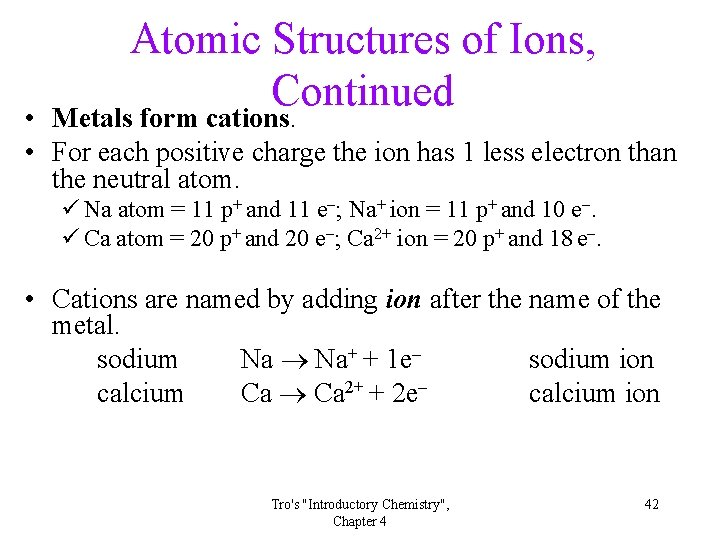Atomic Structures of Ions, Continued Metals form cations. • • For each positive charge the ion has 1 less electron than the neutral atom. ü Na atom = 11 p+ and 11 e ; Na+ ion = 11 p+ and 10 e. ü Ca atom = 20 p+ and 20 e ; Ca 2+ ion = 20 p+ and 18 e. • Cations are named by adding ion after the name of the metal. sodium Na Na+ + 1 e sodium ion calcium Ca 2+ + 2 e calcium ion Tro's "Introductory Chemistry", Chapter 4 42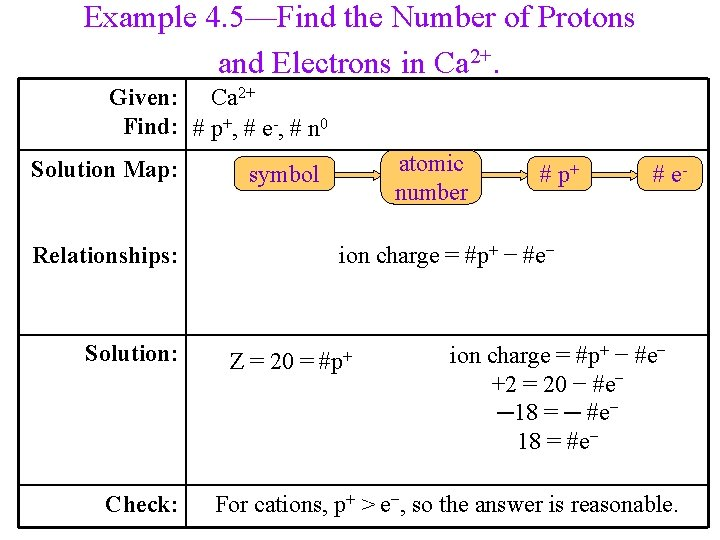Example 4. 5—Find the Number of Protons and Electrons in Ca 2+. Given: Ca 2+ Find: # p+, # e-, # n 0 Solution Map: Relationships: Solution: Check: atomic number symbol # p+ # e- ion charge = #p+ − #e− Z = 20 = #p+ ion charge = #p+ − #e− +2 = 20 − #e− ─18 = ─ #e− 18 = #e− For cations, p+ > e−, so the answer is reasonable.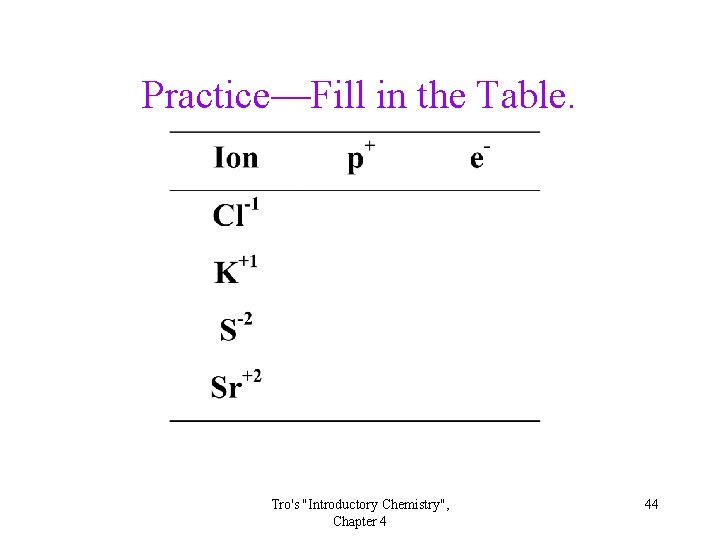Practice—Fill in the Table. Tro's "Introductory Chemistry", Chapter 4 44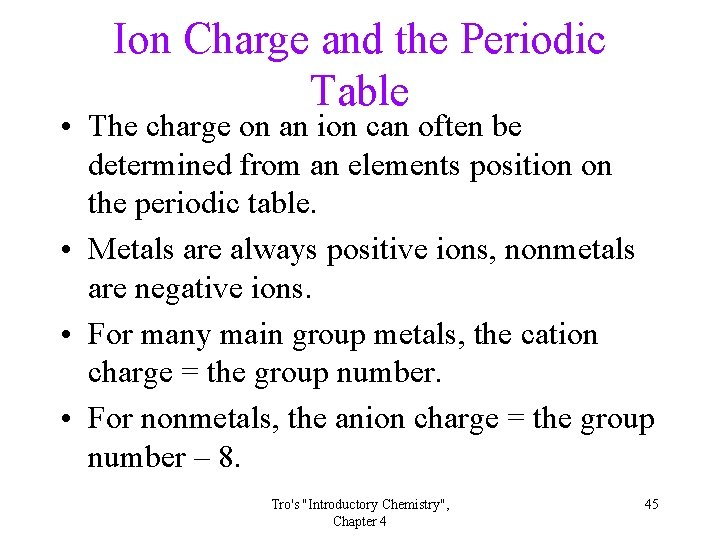Ion Charge and the Periodic Table • The charge on an ion can often be determined from an elements position on the periodic table. • Metals are always positive ions, nonmetals are negative ions. • For many main group metals, the cation charge = the group number. • For nonmetals, the anion charge = the group number – 8. Tro's "Introductory Chemistry", Chapter 4 45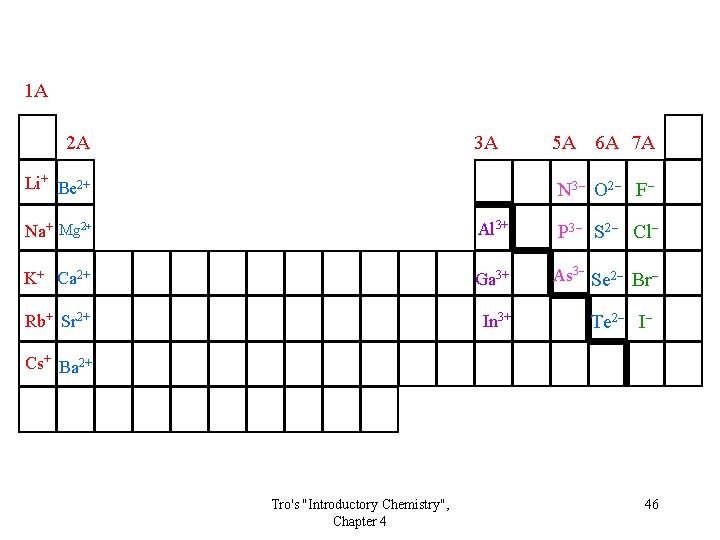1 A 2 A 3 A Li+ Be 2+ 5 A 6 A 7 A N 3 O 2 F Na+ Mg 2+ Al 3+ P 3 S 2 Cl K+ Ca 2+ Ga 3+ As 3 Se 2 Br Rb+ Sr 2+ In 3+ Te 2 I Cs+ Ba 2+ Tro's "Introductory Chemistry", Chapter 4 46Structure of the Nucleus • It is possible for the same element to have atoms with different masses, üSuch elements are called isotopes. üThere are two isotopes of chlorine found in nature, one that has a mass of about 35 amu and another that weighs about 37 amu. • The observed mass is a weighted average of the weights of all the naturally occurring atoms. üThe atomic mass of chlorine is 35. 45 amu. Tro's "Introductory Chemistry", Chapter 4 47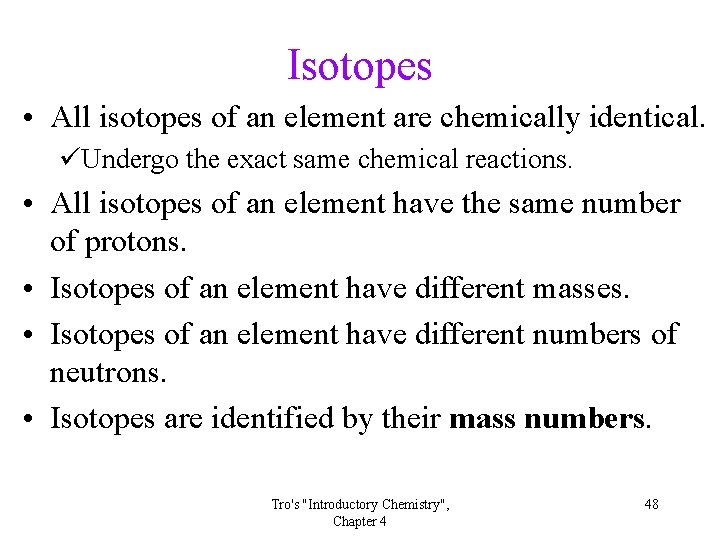Isotopes • All isotopes of an element are chemically identical. üUndergo the exact same chemical reactions. • All isotopes of an element have the same number of protons. • Isotopes of an element have different masses. • Isotopes of an element have different numbers of neutrons. • Isotopes are identified by their mass numbers. Tro's "Introductory Chemistry", Chapter 4 48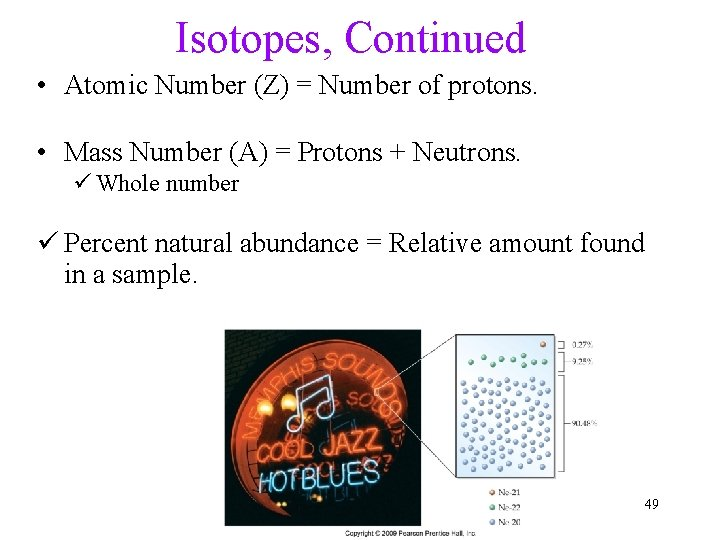Isotopes, Continued • Atomic Number (Z) = Number of protons. • Mass Number (A) = Protons + Neutrons. ü Whole number ü Percent natural abundance = Relative amount found in a sample. 49Isotopes of Chlorine • Cl-35 makes up about 75% of chlorine atoms in nature, and Cl-37 makes up the remaining 25%. • Cl-35 has a mass number = 35, 17 protons and 18 neutrons ( i. e. , 35 - 17). • Cl-37 has a mass number = 37, 17 protons and 20 neutrons ( i. e. , 37 - 17) • The average atomic mass of Cl is 35. 45 amu. AX = Z X-A Atomic symbol A = Mass number Z = Atomic number Tro's "Introductory Chemistry", Chapter 4 Cl or Cl-35 35 17 50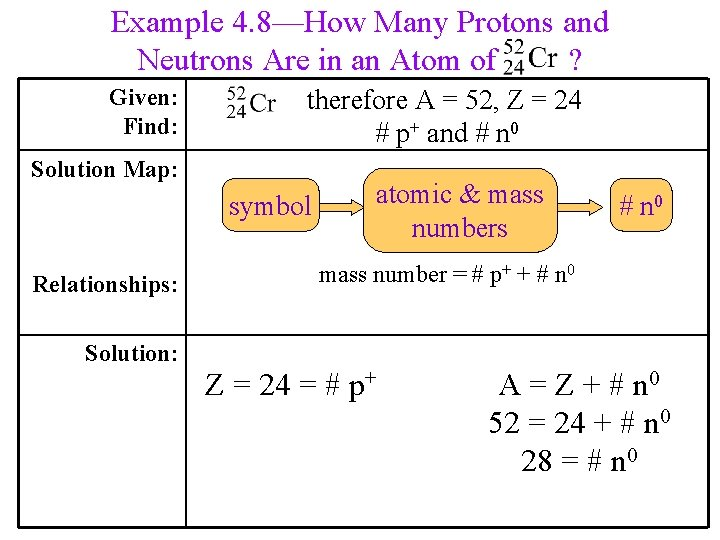Example 4. 8—How Many Protons and Neutrons Are in an Atom of ? Given: Find: therefore A = 52, Z = 24 # p+ and # n 0 Solution Map: symbol Relationships: Solution: atomic & mass numbers # n 0 mass number = # p+ + # n 0 Z = 24 = # p+ A = Z + # n 0 52 = 24 + # n 0 28 = # n 0Practice—Complete the Following Table. Tro's "Introductory Chemistry", Chapter 4 52Isotopes of Neon Symbol Number of A, mass protons neutrons number Percent natural abundance Ne-20 or 10 10 20 90. 48% Ne-21 or 10 11 21 0. 27% Ne-22 or 10 12 22 9. 25% Tro's "Introductory Chemistry", Chapter 4 53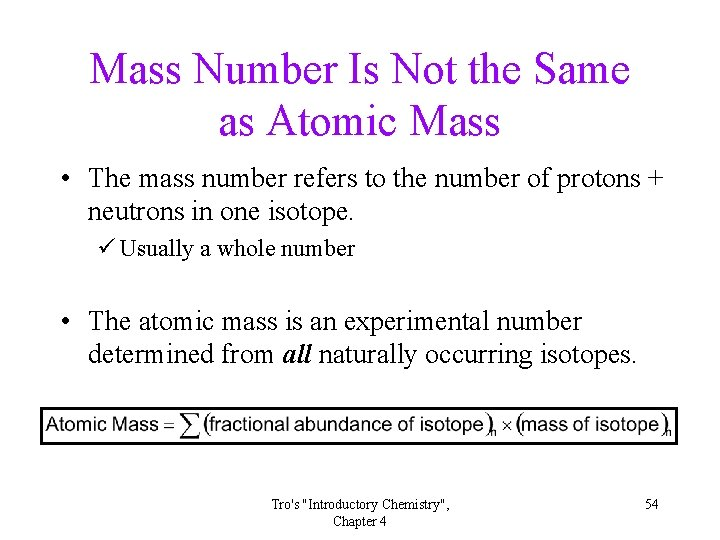Mass Number Is Not the Same as Atomic Mass • The mass number refers to the number of protons + neutrons in one isotope. ü Usually a whole number • The atomic mass is an experimental number determined from all naturally occurring isotopes. Tro's "Introductory Chemistry", Chapter 4 54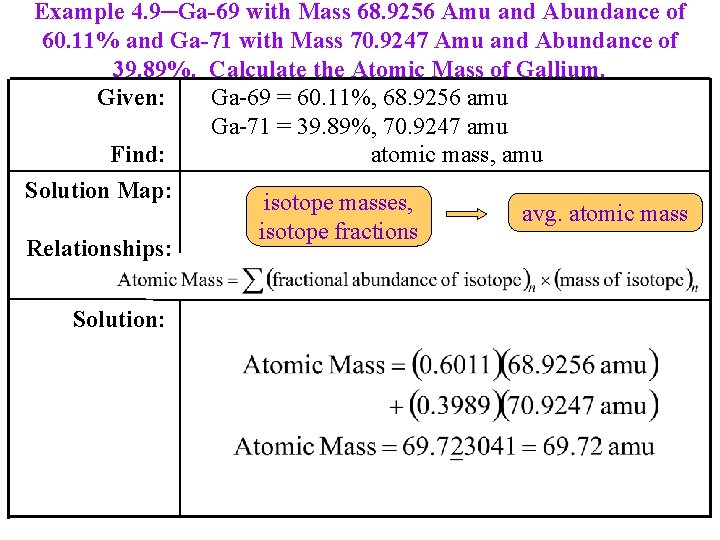Example 4. 9─Ga-69 with Mass 68. 9256 Amu and Abundance of 60. 11% and Ga-71 with Mass 70. 9247 Amu and Abundance of 39. 89%. Calculate the Atomic Mass of Gallium. Given: Ga-69 = 60. 11%, 68. 9256 amu Ga-71 = 39. 89%, 70. 9247 amu Find: atomic mass, amu Solution Map: isotope masses, avg. atomic mass isotope fractions Relationships: Solution:Practice-An element has three naturally occurring isotopes. Their masses and percent natural abundance are given in the table below. Calculate the atomic mass of the element and identify this element. Isotope Atomic mass Percent natural abundance Isotope 1 20 90. 4 % Isotope 2 21 0. 27 % Isotope 3 22 9. 25 % Tro's "Introductory Chemistry", Chapter 4 56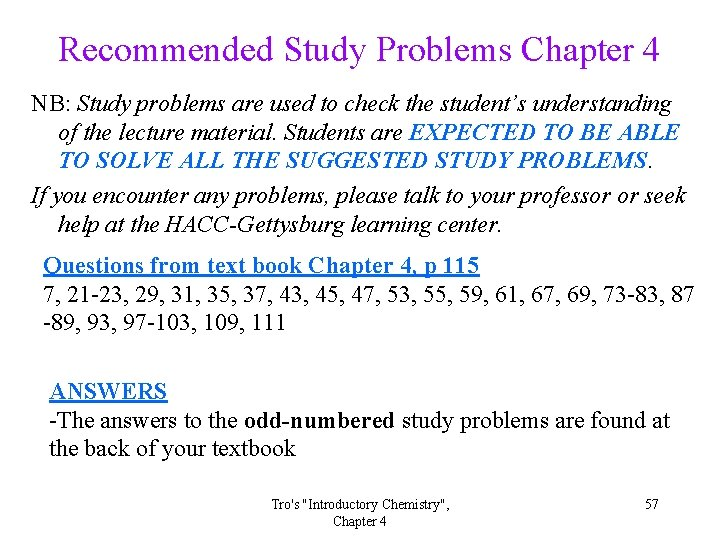Recommended Study Problems Chapter 4 NB: Study problems are used to check the student’s understanding of the lecture material. Students are EXPECTED TO BE ABLE TO SOLVE ALL THE SUGGESTED STUDY PROBLEMS. If you encounter any problems, please talk to your professor or seek help at the HACC-Gettysburg learning center. Questions from text book Chapter 4, p 115 7, 21 -23, 29, 31, 35, 37, 43, 45, 47, 53, 55, 59, 61, 67, 69, 73 -83, 87 -89, 93, 97 -103, 109, 111 ANSWERS -The answers to the odd-numbered study problems are found at the back of your textbook Tro's "Introductory Chemistry", Chapter 4 57